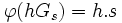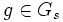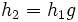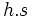# Fundamental theorem of group actions

## Name

This result is also sometimes termed Burnside's theorem.

## Statement

### Statement for transitive group actions

Suppose a group$G$ acts transitively on a nonempty set$S$. Suppose$s \in S$ is a point, and$G_s$ denotes the isotropy subgroup of$s$ in$G$, i.e.:$G_s = \{ g \in G \mid g.s = s \}$.

Then, there exists a unique bijective map between the left coset space of$G_s$ in$G$ and the set$S$:$\varphi:G/G_s \to S$

satisfying the property that it is$G$-equivariant with respect to the natural action on the left coset space; in other words, for any$g \in G$ and any left coset$hG_s$, we have:$\varphi(ghG_s) = g.\varphi(hG_s)$.

Note that this yields:$|G/G_s| = |S|$.

Combining this with Lagrange's theorem, we obtain that:$|G| = |G_s||S|$.

### Statement for more general group actions

Suppose$G$ is a group acting on a set$S$. Let$x \in S$, and$K$ be the orbit of$x$ under the action of$G$. Then, if$G_x$ denotes the stabilizer of$x$ in$G$, we have a bijection:$G/G_x \to K$.

Thus:$|G/G_x| = |K|$

and$|G| = |G_x||K|$

Note that this follows directly from the statement about transitive group actions, because the action of$G$ restricted to the orbit of$x$ is transitive.

## Proof

### Construction of the map

We first describe the map$\varphi$.

For a left coset$hG_s$, define:$\varphi(hG_s) = h.s$.

We need to prove that this is well-defined, and independent of the choice of coset representative. Thus, suppose that$h_1, h_2$ are in the same left coset of$G_s$. Then, there exists$g \in G_s$ such that$h_2 = h_1g$. Thus:$h_2.s = (h_1g).s = h_1.(g.s) = h_1.s$

proving that the map is well-defined and independent of the choice of coset representative.

### Proof that the map is injective

Suppose$h_1,h_2 \in G$ are such that$h_1.s = h_2.s$. Then, applying$h_1^{-1}$ to both sides yields:$h_1^{-1}.(h_1.s) = h_1^{-1}.h_2.s \implies s = (h_1^{-1}h_2).s$

Thus,$h_1^{-1}h_2 \in G_s$, forcing$h_1,h_2$ to be in the same left coset of$G_s$. Thus, two elements from different left cosets cannot send$s$ to the same element.

### Proof that the map is surjective

Since the action of$G$ on$S$ is transitive, every element of$S$ is expressible as$h.s$ for some$h \in G$, and hence as$\varphi(hG_s)$.

### Proof that the map is equivariant

We need to prove that:$\varphi(ghG_s) = g.\varphi(hG_s)$.

The left side is$gh.s$ while the right side is$g.(h.s)$. The two are clearly equal, by the definition of a group action.